Next: Connection between Trees and Up: Methods Previous: Traveling Salesman Problem Application

## Scoring of MSAs without evolutionary trees

The CS algorithm provides a method to evaluate a specific MSA or to compare different algorithms for constructing MSAs. To do so, we abandon scores obtained pairwise from OPAs. Instead, the pairwise relationship between two sequences in the protein family are extracted from the MSA itself. These are scored using a Dayhoff matrix without dynamic programming re-alignment to give an optimal alignment. These are MSA-derived pairwise alignments (MPA).

Definition 2.5   The function S(x, y) scores two symbols: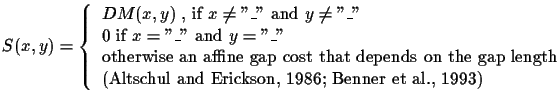DM is an entry in a Dayhoff matrix [Schwarz and Dayhoff, 1979,Gonnet et al., 1992].

The function S(x, y) (see Definition 2.5) scores two symbols x, y that are either amino acids or gap characters in our case.

Definition 2.6   The induced MSA-derived pairwise alignment MPA(ai, ai) of two sequencesis: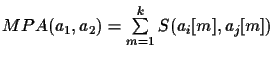MPA scores must be equal to or less than the scores obtained from the OPA pairwise alignments. The CS method can be applied now again without the need for an explicitly defined evolutionary tree by repeating the procedure outlined above, but using MPA scores instead of OPA scores. The CS score of the MSA is the sum of MPA scores in the circular order C divided by two:

Definition 2.7   The score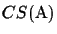of an MSAis defined as: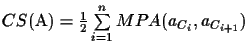where Cn+1 = C1.

Note that if there is a deletion in both sequences, there is no penalty (score is zero) because that deletion happened in some ancestor, and its penalty has been counted already 2.

Lemma 2.4 (Upper Bound)   Let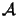be the set of all possible MSAs that can be generated for a given set of sequences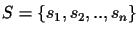. The maximal score CSmax(S) serves as an upper bound for the score of any MSAthat can be built from S: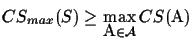Proof 2.4   OPA scores are optimal. Therefore, CSmax(S), which is the sum of OPA scores in circular order obtained by a TSP algorithm, is also maximal. Assume this last statement is wrong there exists another score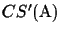that is higher than CSmax(S). Since that scoreis a sum of MPA scores, it would follow that at least one of the MPA scores is higher than the corresponding OPA score, which is a contradiction.

Whenever we score an MSA, regardless of the algorithm used to generate it, the score of this alignment must be less than or equal to the upper bound CSmax(S). Hence the upper bound can be used to evaluate a given MSA. The closer the score of a calculated MSA is to the upper bound, the better the MSA is.
Note that in many cases, as in the following example and always when none of the optimal pairwise alignments of S have gaps, then the score of the MSA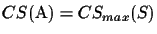.
Example 2.2:  The table to the right in Figure 9 shows the pairwise MPA scores of the sequences from the MSA on the left. It is easy to verify that the longest tour is (A, B, C, D, E, A) and that it is a circular tour in the corresponding tree (see Figure 1). The score of the MSA is the sum of pairwise alignments in circular order divided by two, so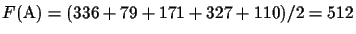. Here the MSA has the optimal score CSmax(S)..

Figure 9: An MSA and a table containing the pairwise scores


A: RPCVCP___VLRQAAQ__QVLQRQIIQGPQQLRRLF_AA
B: RPCACP___VLRQVVQ__QALQRQIIQGPQQLRRLF_AA
C: KPCLCPKQAAVKQAAH__QQLYQGQLQGPKQVRRAFRLL
D: KPCVCPRQLVLRQAAHLAQQLYQGQ____RQVRRAF_VA
E: KPCVCPRQLVLRQAAH__QQLYQGQ____RQVRRLF_AA


 A B C D E A 0 336 27 44 110 B 336 0 79 56 99 C 27 79 0 171 176 D 44 56 171 0 327 E 110 99 176 327 0Next: Connection between Trees and Up: Methods Previous: Traveling Salesman Problem Application
Chantal Korostensky
1999-07-14